# ST_Touches

## Name

ST_Touches — Returns ``` TRUE ``` if the geometries have at least one point in common, but their interiors do not intersect.

## Synopsis

``` boolean ST_Touches ( ``` geometry g1 , geometry g2 ``` ) ``` ;

## Description

Returns ``` TRUE ``` if the only points in common between ``` g1 ``` and ``` g2 ``` lie in the union of the boundaries of ``` g1 ``` and ``` g2 ``` . The ``` ST_Touches ``` relation applies to all Area/Area, Line/Line, Line/Area, Point/Area and Point/Line pairs of relationships, but not to the Point/Point pair.

In mathematical terms, this predicate is expressed as: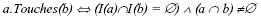The allowable DE-9IM Intersection Matrices for the two geometries are:

• FT*******

• F**T*****

• F***T****Do not call with a ``` GEOMETRYCOLLECTION ``` as an argumentThis function call will automatically include a bounding box comparison that will make use of any indexes that are available on the geometries. To avoid using an index, use ``` _ST_Touches ``` instead.This method implements the OpenGIS Simple Features Implementation Specification for SQL 1.1. s2.1.1.2 // s2.1.13.3This method implements the SQL/MM specification. SQL-MM 3: 5.1.28

## Examples

The ``` ST_Touches ``` predicate returns ``` TRUE ``` in all the following illustrations.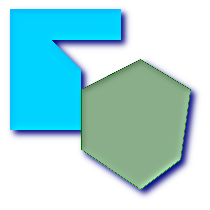``` POLYGON ``` / ``` POLYGON `````` POLYGON ``` / ``` POLYGON ```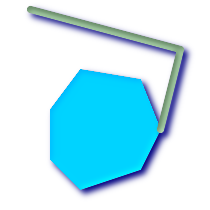``` POLYGON ``` / ``` LINESTRING ```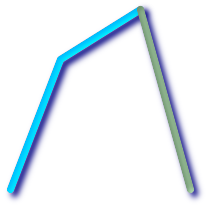``` LINESTRING ``` / ``` LINESTRING `````` LINESTRING ``` / ``` LINESTRING ```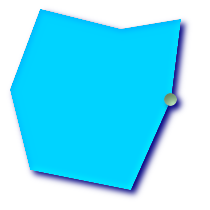``` POLYGON ``` / ``` POINT ```
```SELECT ST_Touches('LINESTRING(0 0, 1 1, 0 2)'::geometry, 'POINT(1 1)'::geometry);
st_touches
------------
f
(1 row)

SELECT ST_Touches('LINESTRING(0 0, 1 1, 0 2)'::geometry, 'POINT(0 2)'::geometry);
st_touches
------------
t
(1 row)```